Complex numbers

This free course is available to start right now. Review the full course description and key learning outcomes and create an account and enrol if you want a free statement of participation.

Free course

# 4.2 Defining useful subsets of the complex number system, and proving the Nested Rectangles Theorem

You will no doubt recall that in real analysis extensive use is made of the modulus function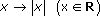. It gives us a way of measuring the “closeness” of two numbers, which we exploit in writing expressions such as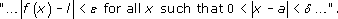We are going to need a similar device in complex analysis and, of course, it is again provided by the modulus function, this time with domain C. In Section 3 we defined |z|, the modulus of the complex number z. The modulus function is the function which maps a complex number to its modulus: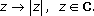Note that the modulus function maps complex numbers to real numbers.

The modulus function with domain C is just an extension of the modulus function that we use in real analysis in the sense that if we restrict its domain to R then the two functions are equal. The value at z of the modulus function, |z|, tells us the distance of the point z from the origin. Similarly, just as |ab| is the distance between the two real numbers a and b, so |zw| is the distance between the two complex numbers z and w.

More explicitly, if z = x + iy and w = u + iv, then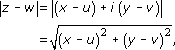which is the usual (Euclidean) distance between the points (x, y) and (u, v).

The function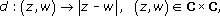is a distance function in the usual sense of the word in that it turns the set of complex numbers into a metric space (see SAQ 33 in Section 4.4). Among other things this means that we have the triangle inequality: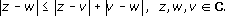(Note that this is an inequality between real numbers (moduli): the modulus function enables us to write inequalities which involve complex numbers.)

This inequality reduces to the usual triangle inequality of real analysis when z, w and v are real, but the two-dimensional nature of complex numbers explains why the triangle inequality is so called. The triangle inequality is as vital to complex analysis as it is to real analysis: it also yields many more inequalities, some of which are in the final problems section, Section 4.4.

In real analysis, the modulus function enables us to describe an interval around a point. For example, we talk of an. neighbourhood of a point x0, as the interval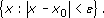The end points of this interval are simply the two elements of the set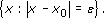In the complex plane the analogous bounding set is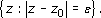which is the set of all points at a distancefrom z0, that is to say the circle centre z0, radius(Figure 25).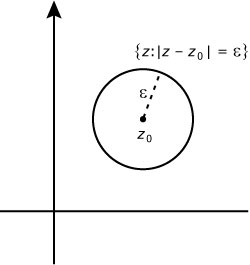Figure 25

Thus in complex analysis, the analogue of an interval is the set of points whose boundary is a circle. Such a set is called a disc. In particular, we make the following definitions.

## Definition

The set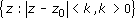is an open disc.

The set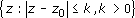is a closed disc.

If we are not sure whether a disc is open or closed, or if we do not want to be specific, or if some but not all points of the bounding circle are included we shall refer simply to the “disc”. We shall also sometimes drop the set notation and write, for example, “the closed disc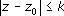” when there is no likelihood of misunderstanding. One other point of convention: we shall refer to the disc centre as the origin and radius 1 as the (open or closed) unit disc.

Two concentric circles specify another type of set of complex numbers – the set of points between the two circles.

## Definition

The set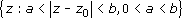is an open annulus.

The set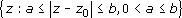is a closed annulus.

If we do not wish to be specific, or if some but not all of the points of the bounding circles are included, we drop the adjective “open” or “closed”. As for discs, we often write, for example, “the open annulus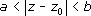”.

Another term which we shall find useful is “bounded set of points”.

## Definition

A set of points is said to be bounded if all points of the set lie in a closed disc with centre the origin.

As an example of the way we can use the modulus function in complex analysis, consider how we can define the limit of a sequence of complex numbers. In real analysis, an infinite sequence is simply a function from the set of natural numbers, N, to R; in complex analysis an infinite sequence is a function from N to C. Just as the terms of a real sequence can be plotted on the number line, so the terms of a complex sequence can be plotted on the complex plane. Convergence is defined precisely as for real sequences.

A sequence {zn} converges to l if for every> 0 there is a natural number N such that, for all natural numbers n,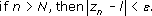The number l is called the limit of the sequence.

In other words, for all n > N every term in the sequence is in the (open) disc centre l radiusNotice that the analytic form of the definition looks just like that of real analysis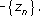converges to l if and only if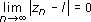— but the geometric interpretation is much more interesting. If the values ofin this definition are made successively smaller, taking values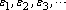then we get a succession of discs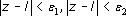, and so on, each disc being a subset of its predecessor and containing its successor. Sequences of sets with this property are often used in complex analysis; they are called nested sequences. They are used to give a method of proof similar to the repeated bisection arguments of real analysis.

Suppose S1, and S2 are two nonempty sets, with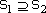. Then there is at least one point common to both sets. Similarly, if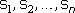are n nonempty sets with– that is to say the sets form a nested sequence – then there is at least one point in common to all n sets. If we try to extend this idea to an infinite sequence of sets then a number of snags can arise which may result in ihe intersection of all the sets being empty.

(i) For example, taking sets in R, suppose Sn is the open interval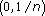. In this case the sets are nested, for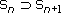for all n, but there is no point in common to every set – the obvious candidate, 0, has been excluded because the intervals are open.

(ii) In other circumstances the “obvious” candidate might be excluded not because the intervals are open but because the sets are subsets of a set which is not complete – Q, the set of rationals, for example. The sets given by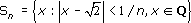are nested but there is no number common to them all. The number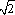is excluded because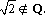(iii) The third snag that might arise with nested sets of real numbers concerns sets such as intervals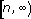. These sets are not open intervals and so are not subject to the first objection. They are also subsets of the complete set, R, and so are not subject to the second objection. But there is no real number in every Sn.

In real analysis, we get over these difficulties by using nested sets that are closed intervals (and therefore subsets of the complete set R) and that are such that the lengths of the intervals have limit 0. There are several ways of extending this idea to C and indeed to metric spaces in general, but a simple and useful way is to extend the idea of an interval to that of a rectangle.

## Definition

The set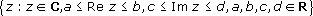is a closed rectangle.

The set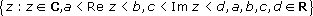is an open rectangle.

(Now look back at SAQ 18 part (v) inSection 3.4.)

If some but not all of the bounding points are included, we shall refer simply to a rectangle and we shall also use this term if we do not wish to be specific.

As a concept analogous to the length of an interval we can use the length of the largest side for a rectangle S. We denote this byℓ(S), so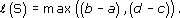Before we propose the Nested Rectangles Theorem we introduce the idea of the intersection of a collection of sets. Suppose that C is a collection of sets and that these sets may be indexed so that we can write C as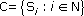We extend the idea of the intersection of two sets to the intersection of the collection of sets in C by regarding the intersection of the Si as the set {x : x is in Si for every iN}; we denote this set by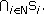In other words,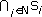denotes the set of elements which are common to all the Si.

We can now propose the Nested Rectangles Theorem.

Theorem 2 (The Nested Rectangles Theorem)

Let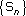be a sequence of nested closed rectangles, with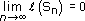, then

(i)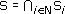is nonempty and contains a unique complex number z0, and

(ii) given> 0, there is an NN such that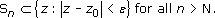(This second part of the theorem tells us that we can always ensure that “eventually” all the rectangles (or, if you prefer, “all but a finite number”) lie inside an arbitrarily small disc centred at z0.)

Proof

(i) Probably the simplest proof of this part is to use the Nested Intervals Theorem from real analysis (see Spivak). If Sn is bounded by the lines Re z = an, Re z = bn, Im z = cn, Im 2  = dn, then the sets In = [ an,bn] form a sequence of nested intervals, and so there is a unique real number a belonging to every In. Similarly, the nested intervals Jn = [ cn,dn] have a unique real number b belonging to all of them. Since Sn = 1n×Jn, the unique number z0 = a + ib belongs to all the Sn and so S contains z0.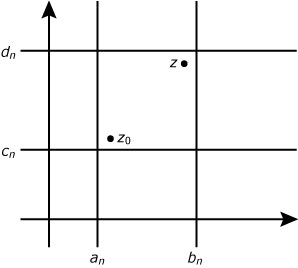Figure 26

(ii) Let zSn. By part (i) z0Sn.

Now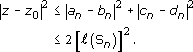But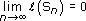; therefore, given> 0, there is a number N such that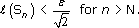Thus, for zSn and n > N,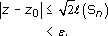In other words,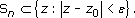M332_1

### Take your learning further

Making the decision to study can be a big step, which is why you'll want a trusted University. The Open University has 50 years’ experience delivering flexible learning and 170,000 students are studying with us right now. Take a look at all Open University courses.

If you are new to university level study, find out more about the types of qualifications we offer, including our entry level Access courses and Certificates.

Not ready for University study then browse over 900 free courses on OpenLearn and sign up to our newsletter to hear about new free courses as they are released.

Every year, thousands of students decide to study with The Open University. With over 120 qualifications, we’ve got the right course for you.

Request an Open University prospectus